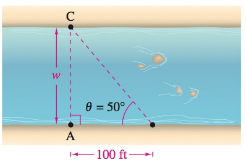Chapter 8.2, Problem 72E### Calculus: An Applied Approach (Min...

10th Edition
Ron Larson
ISBN: 9781305860919

#### Solutions

Chapter
Section### Calculus: An Applied Approach (Min...

10th Edition
Ron Larson
ISBN: 9781305860919
Textbook Problem
1 views

# Width of a River A biologist wants to know the width w of a river in order to set instruments to study the pollutants in the water. From point A, the biologist walks upstream 100 feet and sights to point C. From this sighting it is determined that θ = 50° (see figure). How wide is the river?To determine

To calculate: The width w of the river where the distance between point A and B is 100-foot and the angle between the side AB and BC is 50°.

Explanation

Given Information:

The provided figure is shown below:

The distance between point A and B is 100-foot and the angle between the side AB and BC is 50°.

Formula used:

The definition of trigonometric function Tangent.

tanθ=yx

Where, x is the length of the side adjacent to θ, y is the length of the side opposite to θ, and r=x2+y2 is the length of hypotenuse which is not equal to 0.

Calculation:

Consider the provided figure,

From the figure, the side AB is 100 ft and angle between the side AB and BC is 50°

### Still sussing out bartleby?

Check out a sample textbook solution.

See a sample solution

#### The Solution to Your Study Problems

Bartleby provides explanations to thousands of textbook problems written by our experts, many with advanced degrees!

Get Started

#### Differentiate the function. F(s) = ln ln s

Single Variable Calculus: Early Transcendentals, Volume I

#### (2, 5]

Applied Calculus for the Managerial, Life, and Social Sciences: A Brief Approach

#### Change 125 mm to cm.

Elementary Technical Mathematics

#### ∫x−4dx = −4x−5 + C

Study Guide for Stewart's Single Variable Calculus: Early Transcendentals, 8th